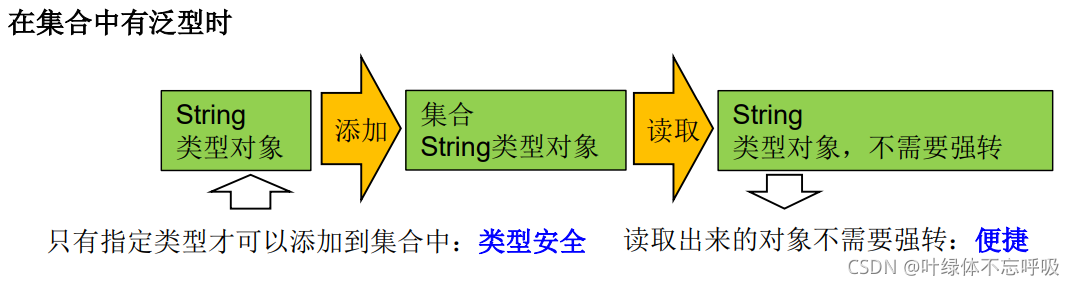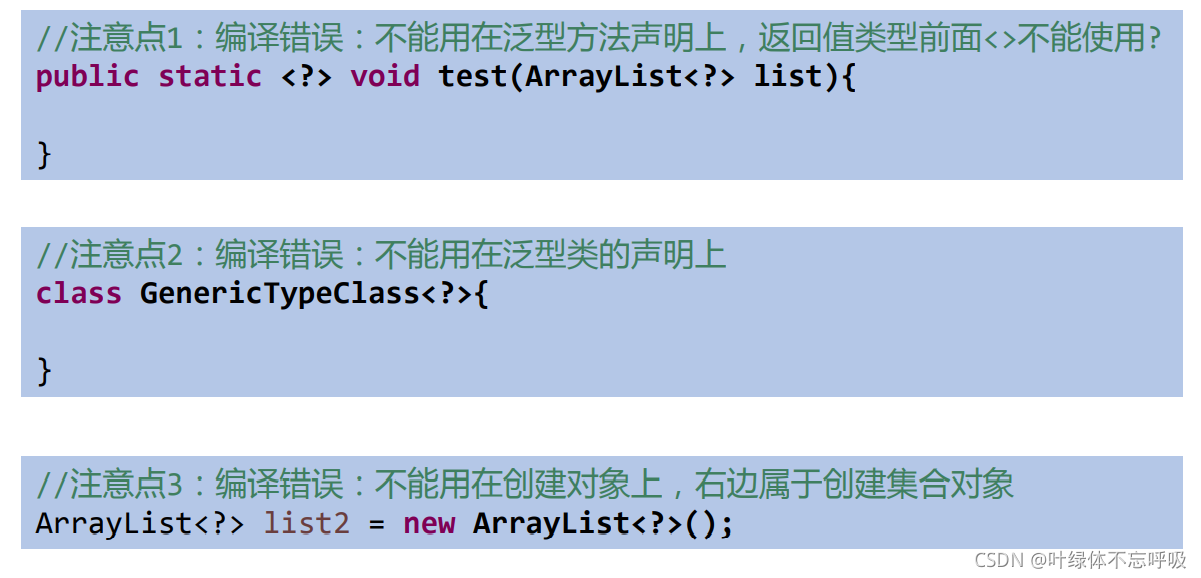# Java泛型在集合使用與自定義及繼承上的體現和通配符的使用

## 泛型的概念## 集合中使用泛型

Collection中使用泛型：

```import java.util.ArrayList;
import java.util.Iterator;

/**
* @Author: Yeman
* @Date: 2021-09-24-15:10
* @Description:
*/
public class GenericTest {
public static void main(String[] args) {
//如下在實例化的時候在<>中填入需要的類型即可（不可以是基本數據類型）
ArrayList<Integer> list = new ArrayList<>();

//遍歷方式一
for (Integer integer : list){
System.out.println(integer);
}
System.out.println("=====================");
//遍歷方式二
Iterator<Integer> iterator = list.iterator();
while (iterator.hasNext()){
System.out.println(iterator.next());
}
}
}
```

Map中使用泛型：

```import java.util.HashMap;
import java.util.Iterator;
import java.util.Map;
import java.util.Set;

/**
* @Author: Yeman
* @Date: 2021-09-24-15:10
* @Description:
*/
public class GenericTest {
public static void main(String[] args) {
//如下如下在實例化的時候在<>中填入需要的類型即可（不可以是基本數據類型）
// 註意因為Map是鍵值對，因此需要分別填入“鍵”和“值”所需要的類型
HashMap<String, Integer> map = new HashMap<>();
map.put("Jack",26);
map.put("Marry",18);
map.put("Tom",20);
map.put("Lily",22);

Set<Map.Entry<String, Integer>> entries = map.entrySet();
Iterator<Map.Entry<String, Integer>> iterator = entries.iterator();
while (iterator.hasNext()){
System.out.println(iterator.next());
}

}
}
```

## 自定義泛型結構

### 1、泛型類、泛型接口

①泛型的聲明
`interface List<T>{}``class GenTest<K,V>{}``class student <T extends Person> {}`

②泛型的實例化

```List<String> strList = new ArrayList<String>();
```
```Iterator<Customer> iterator = customers.iterator();
```

`<>`裡面隻能是類，不能用基本數據類型填充，可以使用包裝類填充。把一個集合中的內容限制為一個特定的數據類型，這就是Generic的核心思想。

`public GenericClass<E>(){}`是錯誤的。

```class Father<T1, T2> {
}
// 子類不保留父類的泛型
// 1)沒有類型 擦除
class Son1 extends Father {// 等價於class Son extends Father<Object,Object>{
}
// 2)指定類型
class Son2 extends Father<Integer, String> {
}
// 子類保留父類的泛型
// 1)全部保留
class Son3<T1, T2> extends Father<T1, T2> {
}
// 2)部分保留
class Son4<T2> extends Father<Integer, T2> {
}
```
```class Father<T1, T2> {
}
// 子類不保留父類的泛型
// 1)沒有類型 擦除
class Son<A, B> extends Father{//等價於class Son extends Father<Object,Object>{
}
// 2)具體類型
class Son2<A, B> extends Father<Integer, String> {
}
// 子類保留父類的泛型
// 1)全部保留
class Son3<T1, T2, A, B> extends Father<T1, T2> {
}
// 2)部分保留
class Son4<T2, A, B> extends Father<Integer, T2> {
}
```
```class Person<T> {
// 使用T類型定義變量
private T info;

// 使用T類型定義一般方法
public T getInfo() {
return info;
}

public void setInfo(T info) {
this.info = info;
}

// 使用T類型定義構造器
public Person() {
}

public Person(T info) {
this.info = info;
}
}
```

### 2、泛型方法

```訪問權限 是否為靜態 <泛型> 返回類型 方法名(泛型標識 參數名稱,...) 異常{
//方法體
}
```
```public class Test {
public <E> E get(int id, E[] arry) {
E result = null;
return result;
}
}
```

## 泛型在繼承上的體現

```ArrayList<String> arrayList = new ArrayList<>();
List<String> list = arrayList;
```## 通配符的使用

### 1、使用

`List<?>``List<String>``List<Object>`等各種泛型List的父類。

```Collection<?> c = new ArrayList<String>();
```
```public static void main(String[] args) {
List<?> list = null;
list = new ArrayList<String>();
list = new ArrayList<Double>();
List<String> l1 = new ArrayList<String>();
List<Integer> l2 = new ArrayList<Integer>();
}
public static void read(List<?> list) {
for (Object o : list) {
System.out.println(o);
}
}
```### 2、有限制的通配符

①<?>

②通配符指定上限
extends：使用時指定的類型必須是繼承某個類，或者實現某個接口，即<=。
`<? extends Number>` (無窮小 , Number]，隻允許泛型為Number及Number子類的引用調用。`<? extends Comparable>`隻允許泛型為實現Comparable接口的實現類的引用調用。

③通配符指定下限

`<? super Number>` [Number , 無窮大)，隻允許泛型為Number及Number父類的引用調用。